# Electrodynamics of Moving Media

The following article is from The Great Soviet Encyclopedia (1979). It might be outdated or ideologically biased.

## Electrodynamics of Moving Media

the branch of electrodynamics that studies electromagnetic phenomena in moving media, particularly the laws governing the propagation of electromagnetic waves. It includes the optics of moving media, which investigates the propagation of light in moving media. Although experimental data on the electrodynamics of moving media had been accumulated over several centuries, a full explanation of the data became possible only after the proposal of the theory of relativity.

The 18th and 19th centuries were marked by the rapid development of Newtonian mechanics, which was used to explain not only the mechanical motion of bodies and continuum dynamics but also thermal phenomena seemingly unrelated to mechanics. The vast majority of physicists were confident that all phenomena in nature could be explained by the action of the laws of classical mechanics. In particular, this belief found expression in the treatment of electromagnetic phenomena. Experiments on the interference of light incontrovertibly demonstrated that light had a wave nature. It was known from mechanics, however, that an elastic medium is needed for the propagation of a wave. It therefore was believed that an elastic medium was also necessary for the propagation of light waves. The vibrations of this light-carrying medium, which was called the ether, were associated with light waves. Since it was known that light also propagates in a vacuum, it had to be assumed that the void was filled with the light ether. The ether was endowed with quite extraordinary properties: on the one hand, it had to have a very high elasticity, since the velocity of light waves is very great (the higher the elasticity of a medium, the greater the wave propagation velocity); on the other hand, it must not offer any mechanical resistance to bodies moving through it, since all bodies move without resistance in a vacuum.

The attempt to explain electromagnetic phenomena by using the ether theory inevitably led to the question of how electromagnetic phenomena occur in a body moving through the ether. The principal theories created in the late 19th century to describe optical phenomena in a moving medium (the theories of H. R. Hertz and H. A. Lorentz) were based on the concept of the ether. They were inconsistent, however, with some experiments known at the time.

The creation of a noncontradictory electrodynamics of moving media became possible after the proposal in 1905 of A. Einstein’s special theory of relativity, which eliminated the ether as the light-carrying medium and as a preferred frame of reference. The concepts of a medium at rest and a moving medium ceased to be absolute and came to be determined only by the selection of a frame of reference (and by the observer associated with it).

In 1908, H. Minowski demonstrated that Maxwell’s equations for media at rest, together with Einstein’s principle of relativity, unambiguously define an electromagnetic field in a moving medium. The same equations can also be derived in a different way—by averaging the microscopic equations of the Lorentz electron theory (seeLORENTZ-MAXWELL EQUATIONS) with allowance for the fact that all parties of a medium have a velocity of ordered motion.

The equations for fields in a moving medium coincide with Maxwell’s equations in a medium at rest: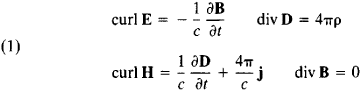Here E and H are the electric field strength and magnetic field strength, respectively, D and B are the electric flux density and magnetic flux density, respectively, and p and j are the densities of external charges and currents, respectively.

The system of equations must be supplemented by constitutive equations, which relate the field strengths to the flux densities. In a medium at rest, the constitutive equations have the form

(1a) D = ∊E B = μH

where ∊ is the dielectric constant of the medium and μ is the magnetic permeability of the medium. The form of these relations in a medium at rest unambiguously yields their form in a medium moving with velocity v: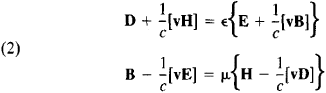(the brackets indicate a vector product). These are Minkowski’s constitutive equations; when v = 0, they become equations (la). The constitutive equations (2), which derive from the principle of relativity, in combination with Maxwell’s equations (1), satisfactorily explain the results of all experiments designed to study electromagnetic phenomena in moving media. Some of the consequences of the theory of the electrodynamics of moving media are examined below.

Propagation of electromagnetic waves in a moving medium. Let an electromagnetic wave propagate in a medium moving with velocity v: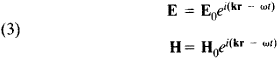Here, E0 and H0 are the amplitudes of the electric and magnetic fields, respectively, k is the wave vector, ω is the angular frequency of the wave, and r and t are the coordinate and time.

It follows from equations (1) through (3) that the wave vector and frequency in a moving medium are related by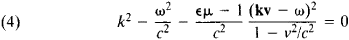When v = 0 (for a medium at rest), we obtain k2 = ∊μω2/c2. Relation (4) includes the angle θ between the direction of propagation of the wave (the vector k) and the velocity v (kv = kv cos θ); the conditions for wave propagation are therefore different for different directions. At small values of v, by confining ourselves to first-order quantities with respect to v/c, it is possible to obtain from (4) the expression for the phase velocity vphase of a wave propagating at an angle θ to the velocity of the medium: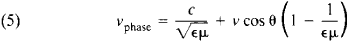The direction of the phase velocity coincides with the direction of the wave vector k. This formula was confirmed in the Fizeau experiment. It is apparent from (5) in particular that the velocity of light in a moving medium is not equal to the sum of the velocity of the medium and the velocity of light in a stationary medium.

The polarization of a wave, that is, the direction of the vectors E0 and H0, depends on the velocity of the medium: the vector E0 is perpendicular not to k, as in a medium at rest, but rather to the vector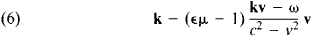which is a linear combination of the velocity of the medium and the wave vector; the vector H0 is not perpendicular to k or E0.

Thus far it has been assumed that the medium moves uniformly and rectilinearly as a whole. If the velocity of the medium is dependent on the cooordinates and time, for example, if the medium is rotating, then the methods of the special theory of relativity become insufficient to determine the electromagnetic field in this case. The form of the field equations can be obtained by means of the general theory of relativity. (The special theory of relativity is applicable at low angular velocities.)

Reflection and refraction of light at moving boundaries. If an electromagnetic wave is incident on the moving boundary of two media, the wave is partially reflected, as in the case of a boundary at rest, and partially passes through the boundary. However, the motion of the boundary leads to several new physical effects. For example, the angle of incidence is not equal to the angle of reflection, and the frequencies of all three waves—incident, reflected, and refracted—are different. There are other differences as well. For example, at certain boundary velocities the reflected wave is absent, and two refracted waves with different frequencies are present.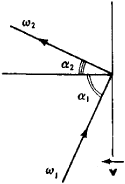Figure 1. Reflection of light from a moving mirror. The angle of reflection α2 Is not equal to the angle of incidence α1, and the frequency ω2 of the reflected light is not equal to the frequency ω1 of the incident light. The mirror is moving with constant velocity v toward the incident light.

Let us examine a very simple example—the reflection of light from a mirror moving in a vacuum (Einstein, 1905). In this case the transmitted wave is absent, and only the incident and reflected waves are present (Figure 1). If the velocity v of the mirror is directed along the normal to the plane of the mirror, and if the wave is incident on the mirror at an angle α, to the normal, then the angle of reflection α2 is expressed in the following way in terms of the angle of incidence: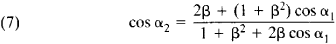where β = v/c (it is assumed that the mirror is moving toward the incident wave). When β = 0 (the mirror is at rest), we have cos α1, = cos α2, that is, the angles of incidence and reflection are equal.

By contrast, if the velocity of the mirror approaches the velocity of light, it follows from (7) that at any angle of incidence the angle of reflection approaches zero—that is, even with glancing incidence the reflected wave moves away from the mirror along the normal. The frequence of the reflected wave is related to the frequency of the incident wave by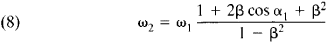If the wave is incident on the moving mirror along the normal, we obtain from (8)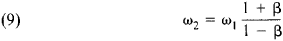If the velocity of the mirror is close to the velocity of light, the frequency of the reflected wave is many times greater than that of the incident wave.

The moving mirror is one example of a moving boundary. In the general case, a boundary is not ideally reflecting, and a refracted wave is therefore present, in addition to the incident and reflected waves. Furthermore, both the boundary and the media on both sides of the boundary may be moving with different velocities. If the velocities of the media on both sides of the boundary are parallel to the boundary the reflection of a wave from such a boundary is accompanied by rotation of the plane of polarization; the angle of rotation is proportional to the relative velocity of the two media.

In order to determine the reflected and refracted waves, one must know the conditions satisfied by the fields at the boundary. In the frame of reference in which the boundary is at rest, the boundary conditions turn out to be the same as in the electrodynamics of stationary bodies.

The velocity of a moving boundary can be determined from the change in frequency when a wave is reflected from the boundary. It has also been proposed to use this effect to multiply the frequency of electromagnetic waves, and it has been suggested that accelerated plasma beams may be used as the reflecting bodies. Experiments have confirmed the feasibility of such an approach, but as yet the efficiency achieved in transforming frequencies is low.

Radiation of electromagnetic waves in a moving medium. Electric charges and currents are the sources of radiation in a moving medium, as in a medium at rest. However, the nature of the propagation of electromagnetic waves from a source located in a moving medium differs substantially from propagation in a medium at rest.

Let the source be located in a moving medium in some small region, and let the radiation time be short. If the medium were at rest, the radiation field would emanate from the source in all directions with identical speeds equal to the speed of light—that is, the entire radiation field would be concentrated near a spherical surface expanding with the speed of light. Motion of the medium causes the speed of light to be different in different directions [see equation (5)]. Therefore, the surface on which the radiation field is not equal to zero is no longer a sphere. Calculation shows that the surface has the form of an ellipsoid of rotation with an axis of symmetry directed along the direction of motion of the medium. The semiaxis of the ellipse increases linearly with time, and the center of the elliptical shell moves parallel to the direction of motion of the medium. Thus, the shell on which the radiation is concentrated simultaneously expands and, in a manner of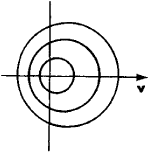Figure 2. Propagation of radiation waves in a moving medium. The radiation source is located at the origin of coordinates. The medium is moving to the right with velocity v. It can be seen that the wave surfaces are “carried along with the flow.” The velocity of the medium does not exceed the phase velocity of light.

speaking, is “carried along with the flow” in the moving medium (is “entrained” by the medium). If the velocity of the medium is comparatively low, the radiation source is located inside the shell (Figure 2). If the rate of motion of the medium exceeds the phase velocity of light, the shell “blows away” so strongly that it turns out to be entirely “downstream,” and the radiation source lies outside the shell (Figure 3).

Passage of a charged particle through a moving medium. In the above examination of radiation in a moving medium, it was assumed that the radiation source was at rest. If the source is in motion, then, as in a medium at rest, the radiation field is determined by the interference of waves emitted by the source at every point on its path. The difference from the case of an isotropic medium at rest is that, because of the effect of entrainment in a moving medium, the speed of waves in different directions is different (see Figures 2 and 3).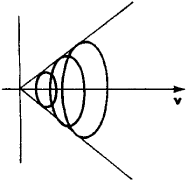Figure 3. Radiation of waves in a moving medium where the velocity of the medium exceeds the phase velocity of light. The radiation source is located at the origin of coordinates. The waves emanating from the source “blow away with the flow” so strongly that they are all situated on the same side of the source.

The distinctive feature of the radiation of a moving source in a moving medium can be understood by using Cherenkov radiation as an example. Let a point charged particle travel with constant velocity u in a medium moving with velocity v. For the sake of simplicity we shall assume that u and v are directed along the same straight line. In the case of a medium at rest (v = 0) a particle may become a radiation source if its velocity is sufficiently great (exceeds the phase velocity of light in the medium,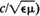). The resulting radiation, called Cherenkov radiation, carries energy away from the moving particle, which therefore decelerates. A slow charged particle or even a charged particle at rest may be a source of Cherenkov radiation in a moving medium. The characteristic wave field, which in this case is Cherenkov radiation, arises if the particle is at rest and if the velocity of the medium exceeds the phase velocity of light. Here, the accelerating force in the direction of motion of the medium acts on the particle that constitutes the radiation source.

The example above shows that in a moving medium the nature of the interaction of a charged particle with the medium is altered. Depending on the velocities of the particle and medium, the energy losses of the particle may differ in magnitude and may even change sign—that is, the particle may be accelerated, rather than decelerated, by the medium.

The production of high-density beams of charged particles moving with relativistic velocity, achieved by means of high-current accelerators and plasma accelerators, has engendered increased interest in the electrodynamics of moving media. In many respects dense beams behave like a macroscopic moving medium. The use of such beams has yielded new possibilities not only in the electrodynamics of moving media in general but also in the study of effects of orders higher than first with respect to v/c—that is, effects in which quantity v/c is no longer small compared with unity.

### REFERENCES

Tamm, I. E. Osnovy leorii elektrichestva, 9th ed. Moscow, 1976.
Tamm, I. E. Sobr. nauchnykh trudov, vol. 1. Moscow, 1975.
Becker, R. Elektronnaia teoriia. Leningrad-Moscow, 1936. (Translated from German.)
Bolotovskii, B. M., and S. N. Stoliarov. “Sovremennoe sostoianie elektrodinamiki dvizhushchikhsia sred (bezgranichnye sredy).” In Einshteinovskii sbornik, 1974. Moscow, 1976.

B. M. BOLOTOVSKII